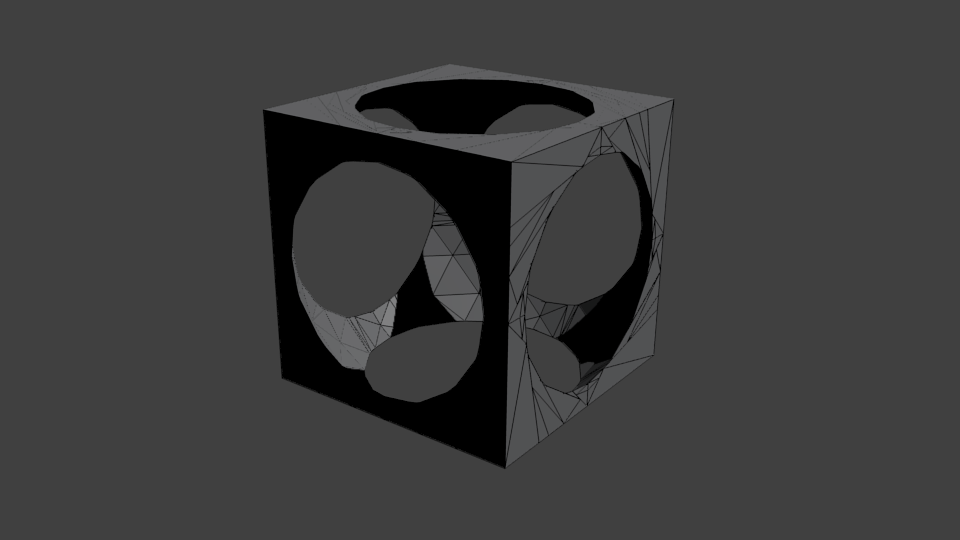## Apache Commons Geometry

Commons Geometry is a general-purpose Java library for geometric processing. The primary goal of the project is to provide a set of geometric types and utilities that are

• mathematically correct,
• numerically accurate,
• easy to use, and
• performant.
Key features of the library include
• Support for Euclidean space in 1, 2, and 3 dimensions
• Support for spherical space in 1 and 2 dimensions
• Support for geometric elements of infinite size
• Support for boolean operations on regions (union, intersection, difference, xor)
• Support for reading and writing common geometric data formats, such as STL and OBJ
• Single external dependency (commons-numbers)

The code below gives a small sample of the API by computing the difference of a cube and an approximation of a sphere and writing the result to a file using the OBJ data format. See the user guide for more details.

// construct a precision instance to handle floating-point comparisons
Precision.DoubleEquivalence precision = Precision.doubleEquivalenceOfEpsilon(1e-6);

// create a BSP tree representing the unit cube
RegionBSPTree3D tree = Parallelepiped.unitCube(precision).toTree();

// create a sphere centered on the origin
Sphere sphere = Sphere.from(Vector3D.ZERO, 0.65, precision);

// subtract a BSP tree approximation of the sphere containing 512 facets
// from the cube, modifying the cube tree in place
tree.difference(sphere.toTree(3));

// compute some properties of the resulting region
double size = tree.getSize(); // 0.11509505362599505
Vector3D centroid = tree.getCentroid(); // (0, 0, 0)

// convert to a triangle mesh
TriangleMesh mesh = tree.toTriangleMesh(precision);

// save as an OBJ file
IO3D.write(mesh, Paths.get("target/cube-minus-sphere.obj"));


Below is an image of the triangle mesh rendered with Blender.